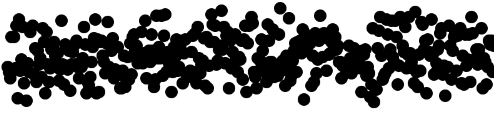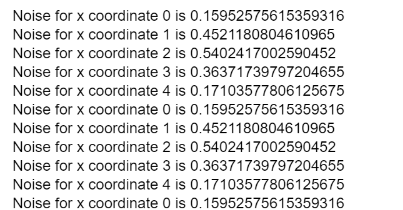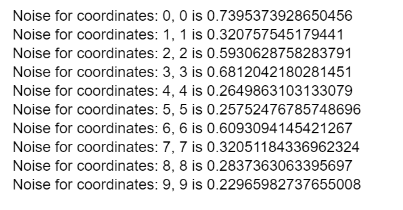Open in App
Not now

# p5.js | noise() Function

• Last Updated : 29 Jan, 2020

The noise() function is used to return a number generated by Perlin noise at the given coordinates. This value is semi-random, which means that the value would be fixed for a coordinate for the lifespan of the program.

The Perlin noise value is different from the value returned by the random() function as this noise has a more natural and harmonic succession compared to the standard one.

Syntax:

`noise(x, [y], [z])`

Parameter: This function accept three parameters as mentioned above and described below:

• x: This is a number which represents the x-coordinate in the noise space.
• y: This is a number which represents the y-coordinate in the noise space. It is an optional parameter.
• z: This is a number which represents the z-coordinate in the noise space. It is an optional parameter.

Return Value: It returns the Perlin noise value between 0 and 1.

Below examples illustrates the noise() function in p5.js:

Example 1: Plotting the noise values of the y-coordinate of the moving point.

• Program:

 `let x_coordinate = 100.0; ` `let plot_x = 10.0; ` `  `  `function` `setup() { ` `    ``createCanvas(400, 200); ` `} ` `  `  `function` `draw() { ` `  `  `    ``// Get noise with x coordinate ` `    ``x_noise = noise(x_coordinate) * 100; ` `  `  `    ``// Plot the point with random noise ` `    ``strokeWeight(10); ` `    ``point(plot_x, x_noise); ` `  `  `    ``// Increment the x coordinate ` `    ``x_coordinate++; ` `  `  `    ``// Increase the x coordinate ` `    ``// for plotting ` `    ``plot_x++; ` `} `

• Output:Example 2: This example demonstrates the semi-random property of a function.

• Program:

 `let x_coordinate = 0.0; ` `let plot_y = 0.0; ` `  `  `function` `setup() { ` `    ``createCanvas(400, 200); ` `} ` `  `  `function` `draw() { ` `     `  `    ``if` `(x_coordinate < 5) { ` `  `  `        ``// Get noise with x coordinate ` `        ``x_noise = noise(x_coordinate); ` `    `  `        ``// Output the noise along with ` `        ``// its corresponding coordinate ` `        ``coord_text = ``"Noise for x coordinate "` `            ``+ x_coordinate + ``" is "` `+ x_noise; ` `         `  `        ``text(coord_text, 10, plot_y); ` `  `  `        ``// Increment the x coordinate ` `        ``x_coordinate++; ` `  `  `        ``// Increase the y coordinate ` `        ``// for plotting ` `        ``plot_y = plot_y + 15; ` `    ``} ` `    ``else` `        ``x_coordinate = 0; ` `} `

• Output:Example 3: This example uses two parameters for defining a point in the noise space.

• Program:

 `let x_coordinate = 0.0; ` `let y_coordinate = 0.0; ` `let plot_y = 0.0; ` `  `  `function` `setup() { ` `    ``createCanvas(400, 200); ` `} ` `  `  `function` `draw() { ` `     `  `    ``if` `(x_coordinate < 10) { ` `  `  `        ``// Get noise with x and y coordinates ` `        ``xy_noise = noise(x_coordinate, y_coordinate); ` `  `  `        ``// Output the noise along with ` `        ``// its corresponding coordinate ` `        ``coord_text = ``"Noise for coordinates: "` `            ``+ x_coordinate + ``", "` `+ y_coordinate ` `            ``+ ``" is "` `+ xy_noise; ` `        ``text(coord_text, 10, plot_y); ` `  `  `        ``// Increment the x and y ` `        ``// coordinates ` `        ``x_coordinate++; ` `        ``y_coordinate++; ` `  `  `        ``// Increase the y coordinate ` `        ``// for plotting ` `        ``plot_y = plot_y + 15; ` `    ``} ` `} `

• Output:Online editor: https://editor.p5js.org/
Environment Setup: https://www.geeksforgeeks.org/p5-js-soundfile-object-installation-and-methods/

Reference: https://p5js.org/reference/#/p5/noise

My Personal Notes arrow_drop_up
Related Articles